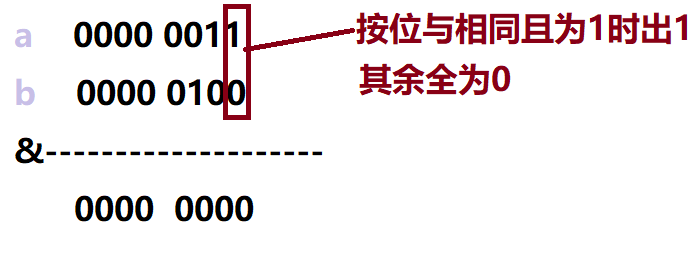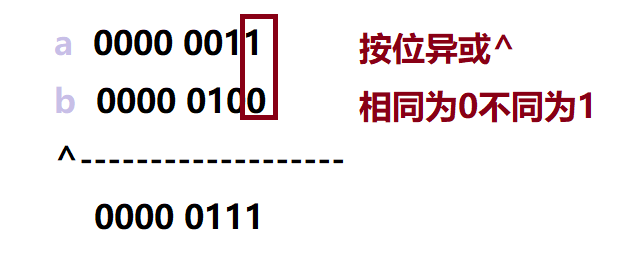# Java之数据类型与运算符详解2845

1

2021年4月7日19:17:33 评论 11 次浏览 4219字阅读14分3秒

# 运算符

char

String 变量名 = 初始值;

char占2个字节！Java中字符都是用Unicode编码

``````public class TestDemo {
public static void main(String[] args) {
float a = 10L;
System.out.println(a);
}
}``````

①float

2.增量运算符:+= -= *= /= %=

long 变量名 = 初始值;//建议数字最后加上大写的L或者小写的l，便于区分。

Java 的 boolean 类型和 int 不能相互转换, 不存在 1 表示 true, 0 表示 false 这样的用法

float 变量名 = 初始值;//建议初始值后加大写F或小写f表示这个数是一个单精度浮点型！

``````public class TestDemo {
public static void main(String[] args) {
int a = 10;
int b =5;
int c =6;
System.out.println(a>b&&a>c);//T
System.out.println(a>b||a>c);//T
System.out.println(a!=b);//T
System.out.println();
System.out.println(a<b&&a>c);//F短路求值，&&运算符中一假为假！
System.out.println(a<b||a>c);//T 或运算符中一真为真！
}
}``````byte 变量名 =初始值;

boolean

int 变量名 = 初始值;short 变量名 = 初始值;char 变量名 = 初始值;

5.字符型变量

short占2个字节

String

<<(左移）：最左侧位不要了，最右侧补0

``````public class TestDemo {
public static void main(String[] args) {
String name1 = "zhangsan";
String name2 = "lisi";
System.out.println(name1+name2);
}
}``````

4.浮点型变量boolean 变量名 = 初始值;

``````public class TestDemo {
public static void main(String[] args) {
int a = 5;
int b = 0;
System.out.println(a/b);
}
}``````5.位运算符

③short

>>（右移）：最右位不要了，正数最左侧补0，负数补1

double 变量名 = 初始值;2.变量的类型

``````public class TestDemo {
public static void main(String[] args) {
long a = 10L;
System.out.println(a);
System.out.println(Long.MAX_VALUE);//long的最大值
System.out.println(Long.MIN_VALUE);//long的最小值
}
}``````

6.移位运算符

1.int 表示变量的类型是一个整型

``````public class TestDemo {
public static void main(String[] args) {
double a = 12.5;
System.out.println(a);
}
}``````

>>>(无符号右移）：最右侧不要了，最左侧补0

# 数据类型

``````public class TestDemo {
public static void main(String[] args) {
int a=1;
int b=a++;//等价于b=a a=a+1此时b=1 a=2;
System.out.println(b);//1
System.out.println(a);//2
b=++a;//等价于a=a+1 b=a 此时a=3 b=3;
System.out.println(b);//3
System.out.println(a);//3
b=a--;//等价于b=a a=a-1 此时b=3 a=2;
System.out.println(b);//3
System.out.println(a);//2
b=--a;//等价于a=a-1 b=a 此时a=1 b=1
System.out.println(b);//1
System.out.println(a);//
}
}``````
``````public class TestDemo {
public static void main(String[] args) {
int a = 5;
a+=1;//等价于a=a+1;此时a=6；
System.out.println(a);//6
a-=1;//等价于a=a-1;上次执行完a+=1时a已经变成了6，这时执行a-=1 a变成了5
System.out.println(a);//5
a*=2;//等价于a=a*2 此时a=10；
System.out.println(a);//10
a/=2;//等价于a=a/2;此时a=5;
System.out.println(a);//5
a%=2;//等价于a=a%2;此时a=1
System.out.println(a);//1
}
}``````

&（按位与） |（按位或）  ~（按位取反） ^（按位异或）

3.整型变量:

1.算术运算符 ：+ - * / %``````public class TestDemo {
public static void main(String[] args) {
int a = 10;
System.out.println(a);
}
}
``````表示int是4个字节 4个字节的范围是[-2^31,2^31-1]

2.变量名是变量的标识后续都是通过这个名字来使用变量

1.首先我们介绍一下什么是变量

3.自增 自减运算符 前缀++ 后缀++ 前缀-- 后缀--表明long 的范围是[-2^63,2^63-1]

代码示例:

​​​​​​​感谢观看！！！

7.条件运算符

②long

``````public class TestDemo {
public static void main(String[] args) {
String name = "zhangsan";
System.out.println(name);
}
}``````

8.字符串类型变量

7.布尔类型变量

①int

<<运算符 相当于 乘2    左移n位  相当于乘2的n次方

Java中可以实现两个字符串"相加",此相加并不是真正的相加,只是将字符串拼接到另一个字符串的后面。

4. 初始化操作是可选的, 但是建议创建变量的时候都显式初始化

``````public class TestDemo {
public static void main(String[] args) {
int a=5;
int b=2;
System.out.println(a+b);//加法运算符结果是7
System.out.println(a-b);//减法运算符结果是3
System.out.println(a*b);//乘法运算符结果是10
System.out.println(a/b);//除法运算符结果是2
System.out.println(a%b);//求余运算符结果是1
}
}``````

``````public class TestDemo {
public static void main(String[] args) {
System.out.println(Integer.MAX_VALUE);//int的最大值
System.out.println(Integer.MIN_VALUE);//int的最小值
}
}``````

4.逻辑运算符：&&（与） ||（或）  ！(非）

``````public class TestDemo {
public static void main(String[] args) {
int a=3;
int b=4;
System.out.println(a&b);//0
System.out.println(a|b);//7
System.out.println(a^b);//7
System.out.println(~a);//-4
}
}``````

long与int对变量命名规则类似，Java中long占8个字节。

3.Java 中 = 表示赋值(和数学不一样), 意思是给变量设置一个初始值

byte

2. 对于 ||, 如果左侧表达式值为 true, 则表达式的整体的值一定是 true, 无需计算右侧表达式.

6.字节型变量

②double

5. 最后不要忘记分号, 否则会编译失败

``````public class TestDemo {
public static void main(String[] args) {
char a ='A';
System.out.println(a);
}
}``````
``````public class TestDemo {
public static void main(String[] args) {
int a = 10;
int b = 2;
int c=a>b?a:b;
System.out.println(c);
}
}``````## JAVA初窥-DAY08

JAVA初窥-DAY08面向过程与面向对象实例化及调用方法和成员变量面向过程与面向对象面向过程：注重的是某件事情过程中的每一个步骤的实现。面向对象：把面向过程中的每一个步骤交给一个...